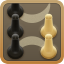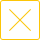# 24点比赛题目大全(包含数字5、6、7的24点比赛题目及答案)

24点比赛题目大全，包含数字5、数字6、数字7的24点比赛题目及答案，24点休闲小游戏适用于家庭游戏、团队建设游戏等，需要准备一副扑克牌，去掉大王、小王、人头牌，只留下数字牌，随机抽出4张，利用加减乘除进行计算，每张牌上的点数使用一次，计算得到24，游戏结束。5555：5*5-5/5=24

5556：5+5*5-6=24

5559：9+5+5+5=24

5566：(5+5-6)*6=24

5567：6+5*5-7=24

5568：8+6+5+5=24

5577：7+7+5+5=24

5578：(5+5-7)*8=24

5588：5*5-8/8=24

5589：8+5*5-9=24

5599：5*5-9/9=24

5666：(5-6/6)*6=24

5667：7+6+5+6=24

5668：6+(8-5)*6=24

5669：6*9-5*6=24

5677：(5-7/7)*6=24

5678：(5+7-8)*6=24

5679：6+(7-5)*9=24

5688：(5+6-8)*8=24

5689：(5+8-9)*6=24

5699：9+(9-6)*5=24

5779：(9-7)*(5+7)=24

5788：8+(7-5)*8=24

5789：(5+7-9)*8=24

5888：5*8-(8+8)=24

5889：(9-5)*8-8=24

6666：6+6+6+6=24

6668：(6+6-8)*6=24

6669：6*6*6/9=24

6679：(6+7-9)*6=24

6688：6/(8-6)*8=24

6689：(6+6-9)*8=24

6789：6*8/(9-7)=24

6799：6*7-(9+9)=24

6888：8+(8-6)*8=24

6889：9*(8+8)/6=24

6899：(9+9)/6*8=24

7889：8+(9-7)*8=24

## 精品排行

1189759人在玩 2
###### 常州四副牌​（四副头）
23201人在玩 3
###### 双陆棋
3## 同城游-本地棋牌游戏合集热门攻略更多